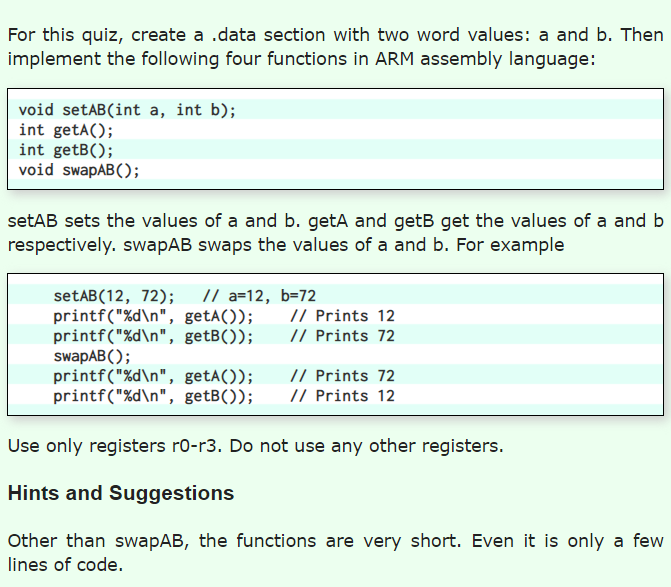Home / Answered Questions / Other / for-this-quiz-create-a-data-section-with-two-word-values-a-and-b-then-implement-the-following-four-f-aw716

# (Solved): For This Quiz, Create A Data Section With Two Word Values: A And B. Then Implement The Following Fou...For this quiz, create a data section with two word values: a and b. Then implement the following four functions in ARM assembly language: void setAB(int a, int b); int getA(); int getB(); void swapAB(); setAB sets the values of a and b. geta and getB get the values of a and b respectively. swapAB swaps the values of a and b. For example setAB(12, 72); // a=12, b=72 printf("%d ", getA()); // Prints 12 printf("%d ", getB()); // Prints 72 swapAB(); printf("%d ", getAO); // Prints 72 printf("%d ", getB()); / Prints 12 Use only registers ro-r3. Do not use any other registers. Hints and Suggestions Other than swapAB, the functions are very short. Even it is only a few lines of code.

We have an Answer from Expert